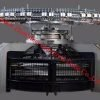# Single Jersey (16-48) dia tube & open, per day capacity 60,000 kg

৳ 12.00

In stock

High productivity pailung machine

Need S/J Order ,Tube &  Open Dia, Free dia mention below

16=4, 17=4, 18=6, 19=6, 20= 3, 22=11, 23=11, 24=9, 25=10,26=4,27=10, 28=6, 29=2, 30=32, 31=14, 32=27, 33=2, 34=23, 36=27, 38=11, 40=9, 42=6, 44=1, 48=3

## Reviews

There are no reviews yet.

## Vendor Information

• Store Name: NRG Hometex Ltd & Composite Yarn Dyeing
• Vendor:
• 3.00 3.00 rating from 1 review
• ## 36 & 38 ‘dia’ 20 ‘g’ jacquard machine 750 kg per day capacity

01
৳ 220.00৳ 230.00

## 36 & 38 ‘dia’ 20 ‘g’ jacquard machine 750 kg per day capacity

01
৳ 220.00৳ 230.00

৳ 20.00

৳ 20.00

৳ 16.00

৳ 16.00
• ## 6 Colour Auto striper S/J (30-40) dia, per day capacity 3200 kg

৳ 90.00৳ 100.00

## 6 Colour Auto striper S/J (30-40) dia, per day capacity 3200 kg

৳ 90.00৳ 100.00৳ 12.00